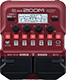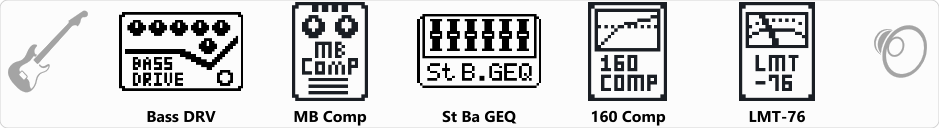# Limeligth

Discussion in 'Zoom B1/B1X Four' started by Adriano Alvares, Jul 18, 2021.

LimeligthDevice: Zoom B1 Four
Firmware: 2.00

Name on device: Limeligth
Optimized for: Phones/Speaker

Effects chain:Limeligth

Effect: "Bass DRV" (Overdrive / Distortion), active - "yes"
"Bass" = 50
"Treble" = 50
"PRSNC" = 75
"Blend" = 70
"Gain" = 30
"VOL" = 76
"MID-F" = 1.0kHz
"MID" = 50

Effect: "MB Comp" (Dynamics), active - "yes"
"Comp" = 70
"LoTHR" = 29
"HiTHR" = 50
"Volume" = 31

Effect: "St Ba GEQ" (Filter), active - "yes"
"50" = 0.0
"120" = 0.0
"400" = 0.0
"500" = 0.0
"800" = 0.0
"4.5k" = 0.0
"10k" = 0.0
"VOL" = 80

Effect: "160 Comp" (Dynamics), active - "yes"
"Threshold" = -22
"Ratio" = 4.0
"Knee" = SOFT
"Volume" = 96

Effect: "LMT-76" (Dynamics), active - "yes"
"Input" = 40
"Ratio" = 4:1
"REL" = 30
"Output" = 35

Patch Volume: 120